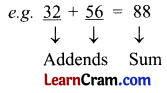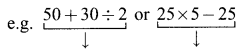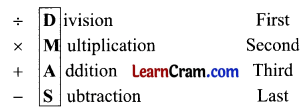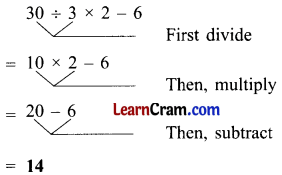# DAV Class 5 Maths Chapter 8 Worksheet 1 Solutions

The DAV Maths Book Class 5 Solutions and DAV Class 5 Maths Chapter 8 Worksheet 1 Solutions of Simplification of Numerical Expressions offer comprehensive answers to textbook questions.

## DAV Class 5 Maths Ch 8 Worksheet 1 Solutions

Question 1.
Simplify the following numerical expressions.
(a) 3 × 2 + 8 – 5
Solution:
3 × 2 + 8 – 5 (3 × 2)
= 6 + 8 – 5 (6 + 8)
= 14 – 5
= 9

(b) 18 + 4 × 6 ÷ 2 – 9
Solution:
18 + 4 × 6 ÷ 2 – 9 (6 ÷ 2)
= 18 + 4 × 3 – 9 (4 × 3)
= 18 + 12 – 9 (18 + 12)
= 30 – 9
= 21(c) 14 + 28 ÷ 7 × 3 – 17
Solution:
14 + 28 ÷ 7 × 3 – 17 (28 ÷ 7)
= 14 + 4 × 3 – 17 (4 × 3)
= 14 + 12 – 17 (14 + 12)
= 26 – 17
= 9

(d) 18 – 2 × 10 ÷ 2 + 2
Solution:
18 – 2 × 10 ÷ 2 + 2 (10 ÷ 2)
= 18 – 2 × 5 + 2 (2 × 5)
= 18 – 10 + 2 (Rearrange)
= 18 + 2 – 10 (18 + 2)
= 20 – 10
= 10

(e) 25 ÷ 5 × 8 + 6 – 12
Solution:
25 ÷ 5 × 8 + 6 – 12 (25 ÷ 5)
= 5 × 8 + 6 – 12 (5 × 8)
= 40 + 6 – 12 (40 + 6)
= 46 – 12
= 34(f) 27 – 9 + 4 × 5
Solution:
27 – 9 + 4 × 5 (4 × 5)
= 27 – 9 + 20 (Rearrange)
= 27 + 20 – 9 (27 + 20)
= 47 – 9
= 38

(g) 17 × 3 + 81 ÷ 9 – 60
Solution:
17 × 3 + 81 ÷ 9 – 60 (81 ÷ 9)
= 17 × 3 + 9 – 60 (17 × 3)
= 51 + 9 – 60 (51 + 9)
= 60 – 60
= 0

(h) 27 – 42 ÷ 3 + 16
Solution:
27 – 42 ÷ 3 + 16 (42 ÷ 3)
= 27 – 14 + 16 (Rearrange)
= 27 + 16 – 14 (27 + 16)
= 43 – 14
= 29DAV Class 5 Maths Chapter 8 Worksheet 1 Notes

Addition, Subtraction, Multiplication, and Division are basic mathematical operations.

When some numbers are added, each number is called an addend. The result obtained is called their sum.Subtraction:

• The large number is called minund.
• The number to be subtracted is called subtrahend.
• The result of subtraction is called difference.

Multiplication:

• The number to be multiplied is called a multiplicand.
• The number with which we multiply is called a multiplier.
• The result after multiplication is called a product.

Division:

• The number to be divided is called a dividend.
• The number by which we divide is called the divisor.
• The number of times the divisor is contained in a dividend is called a quotient.
• After subtracting the product of the divisor and quotient from the dividend, we get the remainder.

Dividend = (Divisor × Quotient) + RemainderA mathematical expression in which two or more operations occur together is called a Numerical Expression.These are called numerical expressions.

Remember: In order to solve a numerical expression we follow the Simplification Rule.Simplify some numerical expressions.

Example 1.
Simplify 20 × 15 – 20
Solution:Example 2.
Simplify 30 ÷ 3 × 2 – 6
Solution: